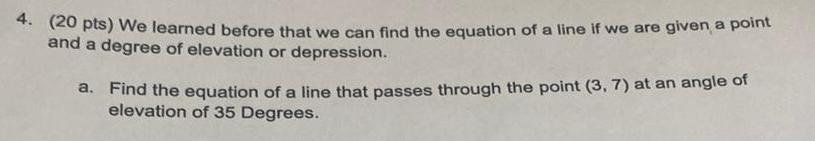Question:

# 4 20 pts We learned before that we can find the equation of

Last updated: 9/16/20234 20 pts We learned before that we can find the equation of a line if we are given a point and a degree of elevation or depression a Find the equation of a line that passes through the point 3 7 at an angle of elevation of 35 Degrees